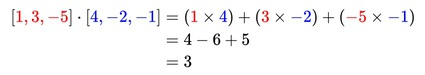### Introduction

In mathematics, the dot product is an algebraic operation that takes two equal-length sequences of numbers (usually coordinate vectors) and returns a single number obtained by multiplying corresponding entries and then summing those products. The name "dot product" is derived from the centered dot " . " that is often used to designate this operation. The dot product is also known as "scalar product", which emphasizes the scalar (rather than vector) nature of the result.

The dot product of two vectors a=[a1,a2,…,an ] and b=[b1,b2,…,bn ] is defined as:In dimension 2, the dot product of vector [a, b] and [c, d] is ac+bd. Similarly, in a dimension 3, the dot product of vector [a, b, c] and [d, e, f] is ad+be+cf.

### Example

The dot product of two three-dimensional vectors [1, 3, -5] and [4, -2, -1] is:### References & Resources

• Wikipedia - http://en.wikipedia.org/wiki/Dot_product